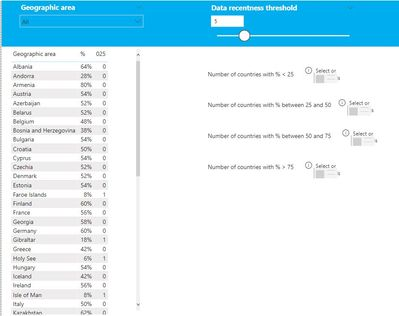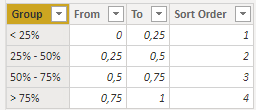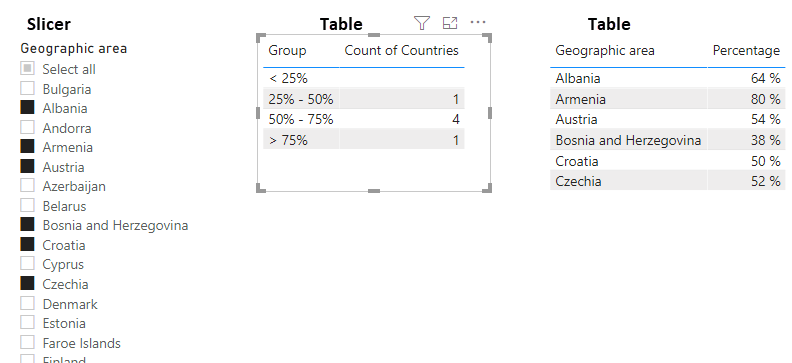cancel
Showing results for
Did you mean:Anonymous
Not applicable

## Calculate Count using a measure that uses moving parameter

Data is mainly indicators and year of publication - table SDG.

I have a table called 'Old' that makes the % of availability change, because it considers only X years old.

On the pbix I upload here I have a page with a table with Country and %.

I need to know:

- Number of countries with % < 25

- Number of countries with % between 25 and 50

- Number of countries with % between 50 and 75

- Number of countries with % > 75

And these numbers will move when the threshold year (Old value) moves, so it has to be a measure.1 ACCEPTED SOLUTIONSuper User

Hey @Anonymous ,

as your measure always needs a filtercontext, you have to create that table internally in the measure and then count the rows with the desired criteria.

The following should work for the 25%:

``````025 =
-- Creates a table with Geographic area and the % measures
VAR vBaseTable =
VALUES ( SDG[Geographic area] ),
"@%", [%]
)
-- Then you filter that table to the rows where % < 0.25
VAR vFilterdTable = FILTER ( vBaseTable, [@%] < 0.25 )
RETURN
-- And then you sum the amount of rows that are left
SUMX ( vFilterdTable, 1 )``````

Then you have to add a second filer criteria for the amount 25-50%:

``````2550 =
VAR vBaseTable =
VALUES ( SDG[Geographic area] ),
"@%", [%]
)
VAR vFilterdTable = FILTER ( vBaseTable, [@%] >= 0.25 && [@%] < 0.5 )
RETURN
SUMX ( vFilterdTable, 1 )``````

And repeat the same for 50-75:

``````5075 =
VAR vBaseTable =
VALUES ( SDG[Geographic area] ),
"@%", [%]
)
VAR vFilterdTable = FILTER ( vBaseTable, [@%] >= 0.5 && [@%] < 0.75 )
RETURN
SUMX ( vFilterdTable, 1 )``````

And last but not least or > 75%:

``````75 =
VAR vBaseTable =
VALUES ( SDG[Geographic area] ),
"@%", [%]
)
VAR vFilterdTable = FILTER ( vBaseTable, [@%] >= 0.75)
RETURN
SUMX ( vFilterdTable, 1 )``````

If you need any help please let me know.
If I answered your question I would be happy if you could mark my post as a solution ✔️ and give it a thumbs up 👍

Best regards
Denis

5 REPLIES 5Anonymous
Not applicable

Thanks for your solutions! I appreciate it!

How would you do to count the empty ones on the 0-25 as well? @selimovd @FrankAT

VAR vFilterdTable = FILTER ( vBaseTable, or([@%] < 0.25, ISBLANK([@%]) )) but it does not seem to work...Super User

Hey @Anonymous ,

that was close. But you have to add it to the vBaseTable as in the filtered the blanks already won't exist.

The following should work:

``````025 =
-- Creates a table with Geographic area and the % measures including blanks
VAR vBaseTable =
VALUES ( SDG[Geographic area] ),
"@%", IF([%] <> BLANK(), [%], BLANK())
)
-- Then you filter that table to the rows where % < 0.25 or BLANK
VAR vFilterdTable =
FILTER ( vBaseTable, [@%] < 0.25 || [@%] = BLANK() )
RETURN
-- And then you sum the amount of rows that are left
SUMX ( vFilterdTable, 1 )``````

If you need any help please let me know.
If I answered your question I would be happy if you could mark my post as a solution ✔️ and give it a thumbs up 👍

Best regards
DenisCommunity Support

Hi @Anonymous ,

Please try to modify your  [Count of INDICATOR not older]:

``````Count of INDICATOR not older = var a=
CALCULATE(DISTINCTCOUNT('SDG'[INDICATOR]), FILTER(SDG,'SDG'[how old] < MAX(Old[Old])+1 )
)
return if(ISBLANK(a),0,a)``````

Then use the following measure:

``````Number of countries with % < 25 = var a =SUMMARIZE(SDG,SDG[Geographic area],"_%",[%]) return COUNTX(FILTER(a,[_%]<=0.25),SDG[Geographic area])+0

Number of countries with %between 25 and 50 = var a =SUMMARIZE(SDG,SDG[Geographic area],"_%",[%]) return COUNTX(FILTER(a,[_%]>0.25&&[_%]<=0.5),SDG[Geographic area])+0

Number of countries with %between 50 and 75 = var a =SUMMARIZE(SDG,SDG[Geographic area],"_%",[%]) return COUNTX(FILTER(a,[_%]>=0.5&&[_%]<0.75),SDG[Geographic area])+0

Number of countries with %between >75 = var a =SUMMARIZE(SDG,SDG[Geographic area],"_%",[%]) return COUNTX(FILTER(a,[_%]>=0.75),SDG[Geographic area])+0

``````

If this post helps, then please consider Accept it as the solution to help the other members find it more quickly.

Best Regards,

Dedmon DaiCommunity Champion

Hi @Anonymous ,

you can do it with a disconnected table which holds the buckets:The report view looks like this:Take a look at the attached PBIX file.Super User

Hey @Anonymous ,

as your measure always needs a filtercontext, you have to create that table internally in the measure and then count the rows with the desired criteria.

The following should work for the 25%:

``````025 =
-- Creates a table with Geographic area and the % measures
VAR vBaseTable =
VALUES ( SDG[Geographic area] ),
"@%", [%]
)
-- Then you filter that table to the rows where % < 0.25
VAR vFilterdTable = FILTER ( vBaseTable, [@%] < 0.25 )
RETURN
-- And then you sum the amount of rows that are left
SUMX ( vFilterdTable, 1 )``````

Then you have to add a second filer criteria for the amount 25-50%:

``````2550 =
VAR vBaseTable =
VALUES ( SDG[Geographic area] ),
"@%", [%]
)
VAR vFilterdTable = FILTER ( vBaseTable, [@%] >= 0.25 && [@%] < 0.5 )
RETURN
SUMX ( vFilterdTable, 1 )``````

And repeat the same for 50-75:

``````5075 =
VAR vBaseTable =
VALUES ( SDG[Geographic area] ),
"@%", [%]
)
VAR vFilterdTable = FILTER ( vBaseTable, [@%] >= 0.5 && [@%] < 0.75 )
RETURN
SUMX ( vFilterdTable, 1 )``````

And last but not least or > 75%:

``````75 =
VAR vBaseTable =
VALUES ( SDG[Geographic area] ),
"@%", [%]
)
VAR vFilterdTable = FILTER ( vBaseTable, [@%] >= 0.75)
RETURN
SUMX ( vFilterdTable, 1 )``````

If you need any help please let me know.
If I answered your question I would be happy if you could mark my post as a solution ✔️ and give it a thumbs up 👍

Best regards
DenisAnnouncements#### Exclusive opportunity for Women!

Join us for a free, hands-on Microsoft workshop led by women trainers for women where you will learn how to build a Dashboard in a Day!#### Power Platform Conference-Power BI and Fabric Sessions

Join us Oct 1 - 6 in Las Vegas for the Microsoft Power Platform Conference.Top Solution Authors
Top Kudoed Authors
Users online (1,716)